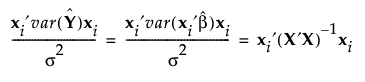Design of Experiments Guide > Technical Details > Relative Prediction Variance
Publication date: 11/29/2021

# Relative Prediction Variance

Consider the following notation:

X

Model matrix. See Model Matrix. Custom designs provide a script that shows the model matrix. See Save X Matrix.

σ2

Error variance.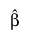Vector of least squares estimates of the parameters.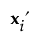The ith row of X.

Using this notation, the predicted response for the ith row of X is given by: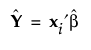The relative prediction variance at the settings defined by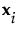is given by: